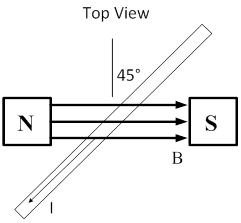### Sample Problem

A wire carrying a 20 A current has a length L = 0.10 m between the poles of a magnet at an angle of 45°, as shown. The magnetic field is uniform and has a value of 0.50 T. What is the magnitude and direction of the magnetic force acting on the wire? N.#### Solution

FB = IlBsinθ =  (20 A)  (0.10 m)  (0.50 T) sin 45°

F = 0.71 N